Home > Mini Projects > Digital Thermometer using 1N4148 Diode

Digital Thermometer using 1N4148 Diode

Abstract - In today ' s world, there is a huge demand for cost-effective and portable thermometers. These thermometers are mainly used for domestic and medical purposes. In this project, we propose a compact and portable design of a digital thermometer for such domestic and medical uses. We have used 1N4148 diode as a temperature sensor and Ngspice as a tool for simulation. The temperature coefficient of the diode, -2 mV/Â°C is exploited for this application to create an accurate digital thermometer. 1N4148 is able to operate as a temperature sensor in the range 0 â„ƒ to 160 â„ƒ. The diode is connected as feedback to an operation amplifier (IC 741), whose output voltage will be a function of temperature. This output voltage will be further amplified by a second operational amplifier and presented as input to an Analog to Digital Converter(ADC). If this thermometer is used in daily life, a digital multimeter/LCD unit will be interfaced to view the reading.

The goal of this project is to propose a device which will combine both these capabilities simultaneously.

In the circuit designed, operational amplifier IC1 (IC 741) provides a constant flow of current through the 1N4148 fast signal silicon diode. The steady state response and the dynamic response of the diode are used to support this statement. The variation in temperature causes the voltage drop across the diode to change. This change must be linear, in order to get reliable results. The output voltage of this IC1 is thus a function of voltage variation across the diode, which in turn is a function of temperature. This output is amplified by second operational amplifier IC2. Preset RV1 is used to set the zero-reading on the meter and preset RV2 is used to set the range of temperature measurement.

I. INTRODUCTION

T HE use of p-n junction characteristics for the design of systems which employ temperature measurement has been done by many researchers. The most prominent among them is Ocaya , who established the linear temperature-voltage relationship for temperature ranges of 0 Â°C to 100 Â°C in a p-n diode, while describing its voltage, current and temperature behaviour. It was also demonstrated by Godwin  that the 1N4148 diode has linear voltage variations with temperature in the range âˆ’40 Â°C to 150 Â°C. The value of the thermal time constant for the diode(1N4148) was found out to be 390 milliseconds. Maximum leakage for both types at 150Â°C is 50 ÂµA at -20V. The property of voltage-temperature relation being linear in temperature range âˆ’40 Â°C to 150Â°C is being extrapolated to make a digital thermometer. The critical requirement of today's world is to have an easily portable temperature sensing instrument, fitted with a convenient display unit, for measuring room and body temperature. Most of the temperature measuring instruments available today are either resistance temperature detectors (RTD), thermocouple digital, or thermistor digital thermometers. The dynamic responses of both diode and a thermocouple was carried out and it was found that diode had a larger thermal time constant than thermocouple. Most of these require a current sensing device either for calibration, or for measuring purposes.

 Output of ADC Display Simulated Unit Circuit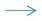Fig.1. Block Diagram I

The expected output voltage range of IC2 op-amp is 0-5 V. This voltage can be given as input to an n-bit Analog to Digital Converter (ADC) which will produce an n-bit digital equivalent corresponding to this voltage. This digital output can be fed to an integrated display unit, or to an LCD / 7-segment display interfaced using embedded systems. [Fig.1.].

 Output of Voltage Multimeter Simulated scaler and (mV) Circuit shifterFig.2. Block Diagram II

Our circuit has one more additional flexibility. Rather than using a separate display unit for displaying the temperature value, the output voltage can be specifically level-shifted and/or scaled so that the numerical value of that scaled voltage in mV is equal to temperature in Â°C. [Fig.2.].

II. CIRCUIT IMPLEMENTATION

Before the hardware implementation of any proposed design, it must be simulated first in software, and checked if it meets our desired specifications or not, whether it gives expected output or not. Based on these results, further course of action will be decided. This project implements the digital thermometer model using Ngspice. Ngspice is a mixed-signal circuit simulator. Spice was originally written at The University of California at Berkeley (USA).To write a Ngspice code, the circuit must first be drawn on project, and all its nodes must be numbered. Then following the syntax as mentioned on Ngspice manual, the components must be included and connected across the nodes. Ngspice implements various elements, like resistors, capacitors, inductors (single or mutual) transmission lines and a number of semiconductor devices like diodes, bipolar transistors, MOSFETs (both bulk and SOI), MESFETs, JFETs and HFETs. Ngspice supports five types of analyses viz. transient, dc, ac, transfer and operating point. Transient analysis includes transient noise simulation. AC analysis includes small-signal noise simulation, pole-zero and transfer function analysis.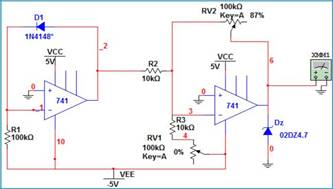Fig.3. Circuit for simulation in Ngspice

NGSPICE NETLIST FOR DIGITAL THERMOMETER:

A. Op-amp IC 741 subcircuit:

*Op amp sub circuit: DM project*

*Op amp sub circuit: DM project*

.SUBCKT OP741 1 2 3 4 5

*nodes: 3=+ 2=- 1=out 5=V+ 4=V-

*Vcc=15 Vee=-15 CC=3e-011 A=200000 RI=2e+006 *Ro=75 VOS=0.001 IOS=2e-008 IBS=8e-008 *VSW+=14 VSW-=-14 CMRR=90

*ISC=0.025 SR=0.5 Fu=1e+006 Pm=6.09112e-007

VC 5 15 DC 1.68573V

VE 12 4 DC 1.68573V

IEE 10 4 DC 1.516e-005A

R1 10 0 10Gohm

R6 11 0 100Kohm

R7 5 4 1Kohm

Rc1 6 5 5305.16ohm

Rc2 5 7 5305.16ohm

Re1 9 10 1839.19ohm

Re2 8 10 1839.19ohm

Ro1 1 14 37.5ohm

Ro2 14 0 37.5ohm

Ree 10 0 1.31926e+007ohm

Rcc 0 13 2.20906e-005ohm

Cee 0 10 1e-012

Cc 14 11 3e-011

C1 6 7 1e-016

GA 11 0 6 7 0.000188496

GC 0 13 1 0 45268.1

GB 14 0 11 0 282.942

GCM 0 11 10 0 5.96075e-009

D1 14 13 Dopamp1

D2 13 14 Dopamp1

D3 1 15 Dopamp2

D4 12 1 Dopamp2

Qt1 6 2 9 Qopamp1

Qt2 7 3 8 Qopamp2

.MODEL Dopamp1 D (Is=7.53769e-014A Rs=0 Cjo=0F Vj=750mV Tt=0s M=0)

.MODEL Dopamp2 D (Is=8e-016A Rs=0 Cjo=0F Vj=750mV Tt=0s M=0)

.MODEL Qopamp1 NPN(Is=8e-016A BF=83.3333 BR=960m

+ Rb=0ohm Re=0ohm Cjs=0F Cje=0F Cjc=0F

+ Vje=750m Vjc=750m Tf=0 Tr=0 mje=0 mjc=0 VA=50)

.MODEL Qopamp2 NPN (Is=8.30948e-016A BF=107.143 BR=960m

+ Rb=0ohm Re=0ohm Cjs=0F Cje=0F Cjc=0F

+ Vje=750m Vjc=750m Tf=0 Tr=0 mje=0 mjc=0 VA=50)

.ENDS

"

B. Digital Thermometer:

.include opampmulti.cir

.model ZENER D(BV=4.7 IBV=5e-3 RS=40 IS=1e-12 )

.MODEL 1N4148 d (

+ IS=6.89131e-09 RS=0.636257 N=1.82683 EG=1.15805

+ XTI=0.518861 BV=80 IBV=0.0001 CJO=9.99628e-13

+ VJ=0.942987 M=0.727538 FC=0.5 TT=4.33674e-09

+ KF=0 AF=1 TNOM=27)

X1 2 1 0 10 9 741

X2 6 3 0 10 9 741

V1 9 0 dc 5V

V2 10 0 dc -5V

R1 1 10 100k

D1 2 1 1N4148

R2 3 2 10k

RV2 3 6 100k

R3 3 4 10k

RV1 4 10 100k

dz 0 6 zener

.dc temp -200 350 1

.control

run

display

set color0=white

set color1=black

set xbrushwidth=3

plot v(6)

plot v(2,1)

meas dc output_v find V(2,1) at =-50

meas dc output_v find V(2,1) at =0 meas dc output_v find V(2,1) at =25 meas dc output_v find V(2,1) at =50 meas dc output_v find V(2,1) at =75 meas dc output_v find V(2,1) at =100 meas dc output_v find V(2,1) at =125 meas dc output_v find V(2,1) at =150 meas dc output_v find V(2,1) at =175 meas dc output_v find V(2,1) at =200 .endc

.end

C. MATLAB code for plot:

clc

close all

clear all

%% DM Project Voltage-Temperature plot for OPAMP I T=-150:1:200;

L=length(T) for i=1:L

"V(i)=-0.0024913*T(i)+0.4886; end

T=-150:1:200; subplot(1,2,1) plot(T,V,'r'); %plotting 11 data points for i=-50:25:200

hold on; plot(T(151+i),V(151+i),'r*');

end

title('Voltage-Temperature Relationship: Stage I');

xlabel('Temperature');

ylabel('Voltage across Diode');

display(L);

%% STAGE II: OPAMP II

%plotting for linear region from -60 deg to 190 deg C

T1=-60:1:190;

L1=length(T1);

for i=1:L1

V1(i)=0.025024962*T1(i)-0.3212851;

end

T1=-60:1:190;

subplot(1,2,2)

plot(T1,V1,'m');

hold on;

%plotting data points for temp: -57,-50,0,50,100,150,182,200

%-57 deg C is min T and 182 is max Temp plot(T1(4),V1(4),'b*'); hold on;

plot(T1(243),V1(243),'b*');

for i=-50:50:190

hold on;

plot(T1(61+i),V1(61+i),'b*');

end

title('Voltage-Temperature Relationship: Stage II');

xlabel('Temperature');

ylabel('Voltage across Diode');

display(L1);

%% ends

III. RESULTS AND DISCUSSION

The simulation results were recorded and analyzed in Ngspice, and the data was plotted using MATLAB and Microsoft Excel for a better view.

A. Variation of diode drop with Temperature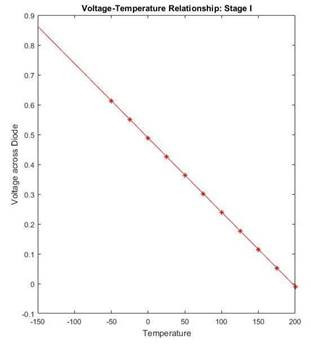Fig.4. Temperature-voltage relationship in the range -

150Â°C to +200Â°C (MATLAB Plot)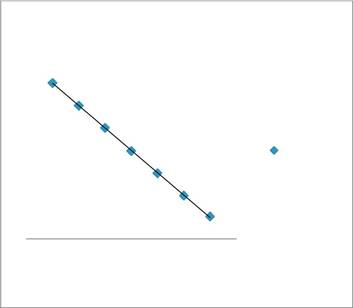Variation of Voltage Drop across Diode

with Temperature

 1 0.9 y = -0.00249x + 0.4886 diode 0.8 RÂ² = 0.9999 0.7 across 0.6 0.5 Voltage Voltage 0.4 0.3 Linear 0.2 (Voltage) 0.1 0 -200 -100 0 100 200

Temperature

Fig.5. Temperature-voltage relationship in the range -

150Â°C to +200Â°C (Excel Plot)

A linear graph of temperature-voltage relationship was obtained. The equation of the graph was found to be

V = -0.00249T + 0.4886, RÂ² = 0.9999

As shown in Fig.5, RÂ² value is very close to unity, showing excellent correlation of the obtained results.

The sensitivity of the diode is

As the output of first op-amp is passed through a second op-amp in inverting mode, hence the temperature-output voltage relationship is also linear, with a positive slope. The equation of the graph was found to be

V=0.02502T - 0.32128, RÂ² = 0.9896

= âˆ’2.49 /Â°This result is a characteristic of the 1N4148 diode.

"

B. Variation of Output Voltage with Temperature

As shown in Fig.7, RÂ² value is very close to unity, showing excellent correlation of the obtained results.

The sensitivity of the device is

= 25.02 /Â°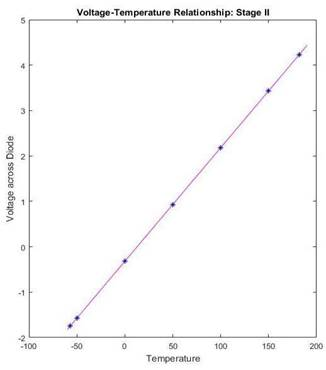Fig.6. Temperature-Output voltage relationship

(MATLAB Plot)

IV. CONCLUSION

We compare the specifications of the device desired, and those met by the simulated device.

Desired Temperature Range = -50Â°C to 200Â°C Desired Output Voltage Range = -2V to 4.5 V

Observed Temperature Range = -57Â°C to 182Â°C Observed Output Voltage Range = -1.747 V to 4.23 V

The observed results are very close to the desired results. An unique device incorporating temperature sensing, RTD calibrating and current measuring in the specified range can be fabricated as shown in Block Diagram II [Fig.2.]